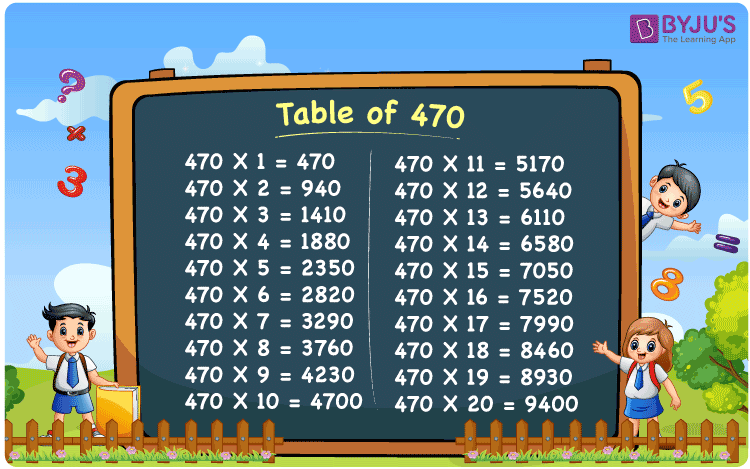Checkout JEE MAINS 2022 Question Paper Analysis : Checkout JEE MAINS 2022 Question Paper Analysis :

# Table of 470

The multiplication of 470 with other numbers is found in the Table of 470. We know that 470 x 10 equals 4700 from the 470 table. Below is a list of multiplication problems of 470 up to the number 20. Students can use the 470 multiplication table to get quick answers. From the link provided here, you can download the Table of 470 in PDF format.

## Table of 470 Chart## What is the 470 Times Table?

The table of 470 up to 20 is given here. Learning the tables provides students with sound understanding, which is important to perform well in their final exams. Using the tables chart as a reference, solving the textbook problems will be easier for the students.

 470×1 = 470 470 470×2 = 940 470 + 470 = 940 470×3 = 1410 470 + 470 + 470 = 1410 470×4 = 1880 470 + 470 + 470 + 470 = 1880 470×5 = 2350 470 + 470 + 470 + 470 + 470 = 2350 470×6 = 2820 470 + 470 + 470 + 470 + 470 + 470 = 2820 470×7 = 3290 470 + 470 + 470 + 470 + 470 + 470 + 470 = 3290 470×8 = 3760 470 + 470 + 470 + 470 + 470 + 470 + 470 + 470 = 3760 470×9 = 4230 470 + 470 + 470 + 470 + 470 + 470 + 470 + 470 + 470 = 4230 470×10 = 4700 470 + 470 + 470 + 470 + 470 + 470 + 470 + 470 + 470 + 470 = 4700

## Multiplication Table of 470

The multiplication table of 470 is given below in tabular form:

 470 × 1 = 470 470 × 2 = 940 470 × 3 = 1410 470 × 4 = 1880 470 × 5 = 2350 470 × 6 = 2820 470 × 7 = 3290 470 × 8 = 3760 470 × 9 = 4230 470 × 10 = 4700 470 × 11 = 5170 470 × 12 = 5640 470 × 13 = 6110 470 × 14 = 6580 470 × 15 = 7050 470 × 16 = 7520 470 × 17 = 7990 470 × 18 = 8460 470 × 19 = 8930 470 × 20 = 9400

## Solved Example on Table of 470

Q. If a person buys 2 oranges per day, how many oranges will he buy in 470 days?

Solution: Given,

Number of oranges a person buys in a day = 2

In 470 days he will buy = 470 x 2

= 940 oranges

## Frequently Asked Questions on the Table of 470

### What is the value of 470 times 11?

From the table of 470, the value of 470 times 11 is 470 x 11 = 5170.

### Determine how many times we should multiply 470 to get 4700.

Using the table of 470, we know that 470 x 10 = 4700. Therefore, we have to multiply 470 ten times to get 4700.

### What is the value of 470 times 15 plus 15 minus 12?

From the table of 470, 470 times 15 plus 15 minus 12
470 x (15 + 15 – 12)
= 470 x 18
= 8460